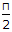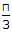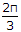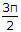# Civil Engineering - Waste Water Engineering

### Exercise :: Waste Water Engineering - Section 2

11.

Sewer ventilating columns are generally placed at

 A. distances 150 m to 300 m B. upper ends of branch sewers C. every change in the size of sewers D. all the above.

Explanation:

No answer description available for this question. Let us discuss.

12.

In case of Imhoff tanks,

 A. the shape is rectangular B. detention period is 2 hours C. the velocity of flow is restricted to 0.30 m/minute D. surface loading is limited to 30, 000 litres/m of plan area per day E. All the above.

Explanation:

No answer description available for this question. Let us discuss.

13.

If the depth of partial flow in a sewer of diameter 2 m, is 50 cm, its wetted perimeter is

 A. π B.C.D.E.Explanation:

No answer description available for this question. Let us discuss.

14.

A drop manhole is provided if

 A. a sewer drops from a height B. a branch sewer joins the main sewer at higher level C. a lamp is inserted to check obstruction D. none of these.

Explanation:

No answer description available for this question. Let us discuss.

15.

In a city the ratio of the drainage to sewage is 20, the percentage discharge passing through non-monsoon periods, is

 A. 5 B. 10 C. 15 D. 20